### NPV Function – Net Present Value – Excel, VBA, G Sheets

This tutorial demonstrates how to use the Excel NPV Function in Excel to calculate the net present value of a series of cash flows.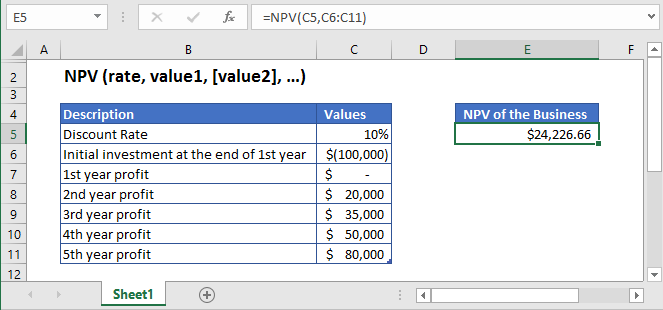## NPV Function Description:

The NPV Function Calculates the net present value.

To use the NPV Excel Worksheet Function, select a cell and type: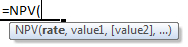(Notice how the formula inputs appear)

### NPV Function Syntax and Inputs:

`=NPV(rate,value1,[value2],...)`

rate – It’s the rate of interest or the discounted rate over one period. It should be entered as a percentage value or a decimal number.

value1 – It’s the numeric values that represent a series of regular cash flows.

value2 – OPTIONAL: Second value(s) representing cash flows.

AutoMacro - VBA Code Generator

## What Is Net Present Value?

The difference between the present value of cash inflows and cash outflows over a specific period is the net present value (NPV). It is assumed that for the investment to be profitable its NPV should be positive and if the NPV is negative then the investment will result in a net loss.

It is simply calculated as: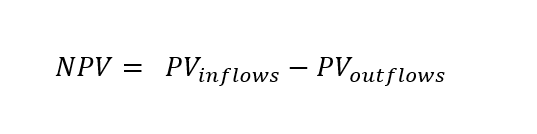## What Is Excel NPV Function?

The Excel NPV function calculates the Net Present Value of an investment based on it’s discounted rate or rate of interest and a series of future cash flows. The Excel NPV Function uses the following equation to calculate the Net Present Value of the investment: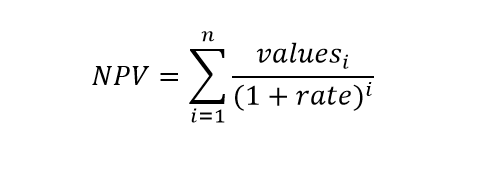Where:

n = number of time periods

i = period number

rate = discount rate or interest rate

values = cash flow

### Calculate Net Present Value of a business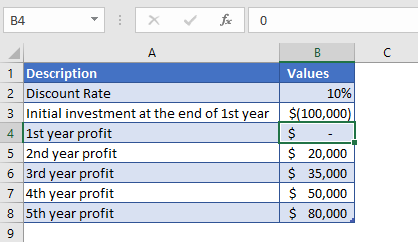Let’s assume, we start a business and the initial investment of \$100,000 will be required at the end of the first year. And it is expected that the business would start generating profit from the second year. The second, third, fourth, and fifth-year profits are projected to be \$20,000, \$35,000, \$50,000, and \$80,000 respectively. The rate of return we could get in alternate investment is 10%. So, we need to find out the net present value of the business.

The initial investment of the business is entered with a negative sign as it represents outgoing cash

Value 1 = -\$100,000

The formula used for the calculation of the net present value of a business is:

`=NPV(B2,B3:B8)`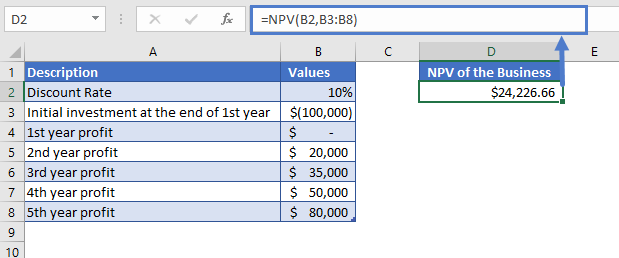The Net Present Value of the business calculated through Excel NPV function is

NPV = \$24,226.66.

### Calculate Net Present Value of a Project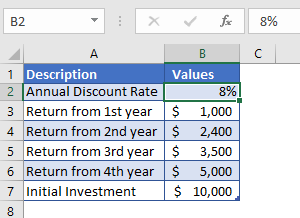Let’s calculate the Net Present Value of a project, where an investment of \$10,000 was made at the start. The annual discount rate is 8%. The returns from 1st year to 4th year are given in the above table.

The formula used for the calculation is:

`=NPV(B2,B3:B6)`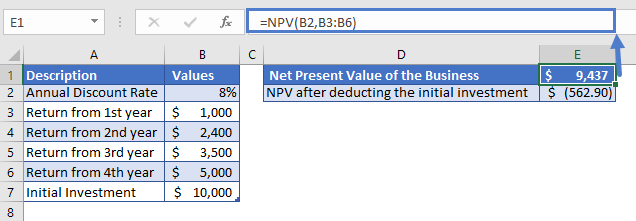The Net Present Value of the business calculated through Excel NPV function is

NPV = \$9,437.1

After deducting the initial investment from the above value, we’ll get a better picture of the investment

NPV (after deducting initial investment) = \$9,437.1 – \$10,000 = -\$562.90

The Net Present Value of the business is -\$562.90.

The NPV result came in negative, it means that the investment would still not be profitable at the end of 4th year.

## NPV in Google Sheets

All of the above examples work exactly the same in Google Sheets as in Excel.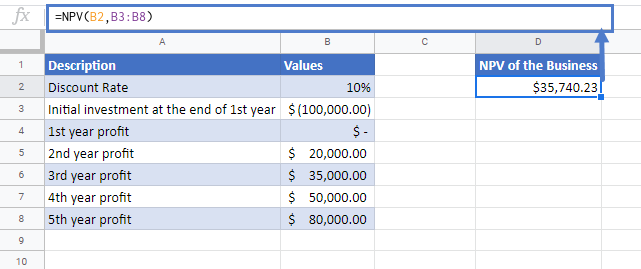## Additional Notes

Use the NPV Function to calculate the Net Present Value of an investment.

Values must be in chronological order. So the payment and income values must be entered in the correct sequence and these values must occur at the end of the period.

In Excel 2007 to 2019, from 1 to 254 value arguments can be entered that represent the payments and income. In excel 2003 and older versions up to 30 value arguments can be entered.

Cash inflows(where cash is received such as coupon payments) have a positive sign while cash outflows (where cash is paid out such as money used to purchase the investment) have a negative sign.

NPV and PV (present value) function are quite similar. The only difference between NPV and PV is that PV allows cash flows to begin at the start of the investment period and also at the end of the investment period.

Periodic cash flows are required. Cash flows most occur on a consistent basis each period.

## NPV Examples in VBA

You can also use the NPV function in VBA. Type:
`application.worksheetfunction.npv(rate,value1,value2)`
For the function arguments (rate, etc.), you can either enter them directly into the function or define variables to use instead.

Return to the List of all Functions in Excel

## Excel Practice Worksheet

Practice Excel functions and formulas with our 100% free practice worksheets!

• Automatically Graded Exercises
• Learn Excel, Inside Excel!